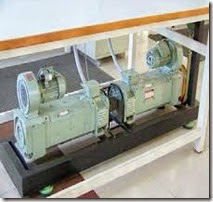# Testing of DC Machines### Introduction:

To judge the performance of a DC machine either Motor or Generator there are several test conducted that are conducted. Mainly one important test named efficiency of a DC machine to be conducted. Since the efficiency depends on the losses of DC machines. If the losses are small then the efficiency of a DC machine is high and vice versa. The deliberation of losses in a DC machine is much important for the 2 principle reasons. The first reason is losses decide the efficiency of the DC machine and substantially impact its working cost. Then another reason is losses conclude the temperature of the DC machine. Therefore machine output power might be obtained without undue weakening of insulation.

### Efficiency of a DC Machine:

The power receives that a DC machine is input and the power which gives out is output. Thus, the DC machine efficiency like that of some energy transferring device is known by:

Efficiency = Output / Input

Input = Output + Losses

Output = Input – Losses

Therefore the DC machine efficiency may also be expressed as:

Efficiency = (Input – Losses) / Input
Or
Efficiency = Output / (Output + Losses)Plugging, Regenerator Braking of DC Motor

To determine the efficiency in a DC machine, using the first equation is the most preferable method to directly load it and measure the input and output power. This method undergoes from three important disadvantages.

• To use this method needs the application of load on the DC machine.
• The loads size may not be available for large size of machines.
• Even though it is possible to give the large size of machine load details, power dissipation will be high and making it an expensive method.

The utmost usual method for computing the efficiency of a DC machine is to determine its losses. For which we need to use the equation 2 or equation 3 to determine the efficiency of a DC machine. This method is the most preferable advantage of suitability and economy.This post first appeared on My Tech Info, please read the originial post: here

# Share the post

Testing of DC Machines

×